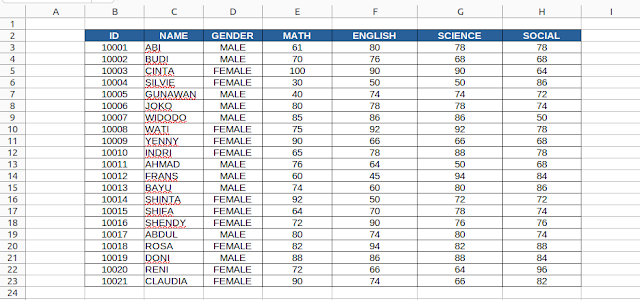Senior author, Open Source enthusiast.
Saturday, July 23, 2022 at 16:09

# LibreOffice Calc Basics VII: VLOOKUP

This is the seventh part of Calc Basics and in this chance we will learn about VLOOKUP formula. For that purpose, we will make a simple student score viewer, so we can select a student's name for the computer to display his/her scores. As a reminder, if you haven't followed this Calc series, read the first and second parts here. Now let's try it.

Subscribe to UbuntuBuzz Telegram Channel to get article updates.

# Dataset

Type this and save the document as calc-07-vlookup.ods. Give the sheet name VLOOKUP. Notice that the table spans across B2:H23. Notice also that the score data spans across E2:H23 from MATH to SOCIAL columns.(The dataset is the same as the previous Calc Basics VI)

Before going to the formula, we should first make a drop down menu for the student name:

1. Put cursor at K2

2. Go to menu Data > Validity > Allow: Cell Range > type Source: \$Sheet1.\$C\$3:\$C\$23 > OK.

Alternatively, click select and drag cells from ABI to CLAUDIA as input to Source. See animation below.

3. Drop down menu created at K2.

4. Try to click the drop down button and select the name ABI.

# VLOOKUP

1. Put cursor at K3

2. Type the formula =VLOOKUP(K2, C3:H23, 3,0)

3. You should get ABI's math score correctly by 61.

4. Put cursor down at K4

5. Type the formula =VLOOKUP(K2, C3:H23, 4,0)

6. You should get ABI's english score correctly by 80.

7. Put cursor down at K5

8. Type the formula  =VLOOKUP(K2, C3:H23, 5, 0)

9. You should get ABI's science score correctly by 78.

10. Put cursor down at K6.

11. Type the formula =VLOOKUP(K2, C3:H23, 6, 0)

12. You should get ABI's social score correctly by 78.

13. Now try to change ABI to any other student and you should see this table displays each student's scores correctly.

(Displaying VLOOKUP formulas)

# Final Result

Below is an example of three VLOOKUP instances displaying three student's sets of scores of ABI, CINTA, and CLAUDIA. You can observe the individual scores match the score table on the left.

To this point, you've learned about basic use of VLOOKUP with example. Next time, we will learn about its counterpart, HLOOKUP. See you next time!